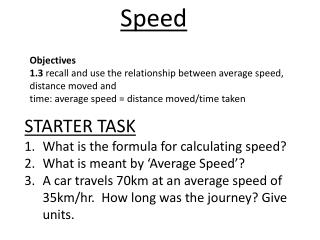Download PresentationSpeed

# Speed

Download Presentation## Speed

- - - - - - - - - - - - - - - - - - - - - - - - - - - E N D - - - - - - - - - - - - - - - - - - - - - - - - - - -
##### Presentation Transcript

1. Speed Objectives 1.3 recall and use the relationship between average speed, distance moved and time: average speed = distance moved/time taken STARTER TASK What is the formula for calculating speed? What is meant by ‘Average Speed’? A car travels 70km at an average speed of 35km/hr. How long was the journey? Give units.

2. What are the units of speed when: Distance is measured in metres and time in seconds Distance is measured in miles and time in hours Distance is measured in kilometres and time in hours Distance is measured in metres and time in minutes Distance is measured in millimetres and time in seconds

3. Speed speed = distance time In physics speed is usually measured in: metres per second (m/s)

4. speed x time also: distance = and: time = distance distance speed speed time

5. 6 = 12 2 What else do you know? 2 = 12 6 12 = 6 x 2 2 = 10 5 What else do you know? 5 = 10 2 10 = 5 x 2 5 = 15 3 What else do you know? 3 = 15 5 15 = 5 x 3

6. Speed = Distance Time What else can you tell me? 2 = 10 5 Based on the above equation, how do I work out distance? Distance = Speed x Time Based on the above equation, how do I work out time? Time = Distance ÷ Speed

7. Usian Bolt ran 100m in 9.58 seconds, what was his average speed? 10.43 m/s (23 mph)

8. Pairs of cameras spot your number plate, start a timer, and then spot your number plate again leaving a zone Question 2 800m The speed cameras record that a car takes 350 seconds to move between them. Calculate the average speed of the car. Show your working. Give the unit. 2.28 m/s

9. Charlie Adam takes a free kick, He is 26 metres away from the goal and it took 1.02 seconds before it crosses the goal line. How fast does the ball travel? 25.49 (metres / second) (x2.24 to get Miles an Hour) = 57 mph

10. http://www.metacafe.com/watch/2755153/matrix_car_chase/

11. Activity 1 Plot these results on a speed time graph, with time on the bottom axis (the x axis) and speed on the side axis (the y axis). Label the points where there is a change in motion A,B,C,D,E,F,H,I,J and K. Example: point A is at time 0 and speed 0.

12. If you walk for 1 1/2 hours at an average speed of 4 miles/h. How far will you have walked? Distance = Speed × Time Distance = 4 × 1.5 = 6 miles How long does it take to drive to Leeds from Blackpool (85 Miles) if you travel at an average speed of 60 mph? Time = Distance ÷ Speed Time = 85 ÷ 60 = 1 Hour and 41 Minutes

13. 1 meter per second = 2.23693629 (2.24) mph. So, take meters per second and multiply by 2.23693629 (2.24) to get miles per hour. Example, 55 meters per second = 123.03 mph.1m/s = 2.2369mph Read more: http://wiki.answers.com/Q/How_do_you_convert_meters_per_second_into_miles_per_hours#ixzz1IZCtHIRh http://www.youtube.com/watch?v=MeCadjzVNZ8

14. Distance, time and speed To work out the speed of an object you need to know: • the distance travelled; • how long it took to travel that distance.

15. Speed calculation example d (distance in km) average speed (in km/h) = t (time in h) d 10 km = 1 h s x t A boy takes 1 hour to travel from his home to the cinema, a distance of 10 km. Calculate his average speed in km/h. = 10 km/h Cover the quantity to be calculated - s (speed)

16. Speed calculation example – units check d d (distance in m) average speed (in m/s) = t (time in s) s x t 10,000 m = 1x60x60 3600 s Sometimes the units have to be changed in a speed calculation. Here is the same problem but with different units: A boy takes 1 hour to travel from his home to the cinema, a distance of 10 km. Calculate his average speed in m/s. = 2.8 m/s Cover the quantity to be calculated - s (speed)

17. Speed calculation – question 1 d s x t Question 1 A group set off from home and walk at an average speed of 3.6 km/h. How far would they travel in two hours? Give your answer in km. distance (km) = speed (km/h) x time (h) = 3.6 km/h x 2 h = 7.2 km

18. Speed calculation – question 1 distance time= speed d 10 km = s x t 5.4 km/h Question 1 How long would it take a woman to walk 10 km if her average speed is 5.4 km/h ? =1.85 hours